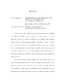## APPROXIMATION ALGORITHMS FOR FACILITY LOCATION AND CLUSTERING PROBLEMS2017
##### Authors
Trinh, Khoa
Facility Location (FL) problems are among the most fundamental problems in combinatorial optimization. FL problems are also closely related to Clustering problems. Generally, we are given a set of facilities, a set of clients, and a symmetric distance metric on these facilities and clients. The goal is to open'' the best'' subset of facilities, subject to certain budget constraints, and connect all clients to the opened facilities so that some objective function of the connection costs is minimized. In this dissertation, we consider generalizations of classical FL problems. Since these problems are NP-hard, we aim to find good approximate solutions in polynomial time. We study the classic $k$-median problem which asks to find a subset of at most $k$ facilities such that the sum of connection costs of all clients to the closest facility is as small as possible. Our main result is a $2.675$-approximation algorithm for this problem. We also consider the Knapsack Median (KM) problem which is a generalization of the $k$-median problem. In the KM problem, each facility is assigned an opening cost. A feasible set of opened facilities should have the total opening cost at most a given budget. The main technical challenge here is that the natural LP relaxation has unbounded integrality gap. We propose a $17.46$-approximation algorithm for the KM problem. We also show that, after a preprocessing step, the integrality gap of the residual instance is bounded by a constant. The next problem is a generalization of the $k$-center problem, which is called the Knapsack Center (KC) problem and has a similar budget constraint as in the KM problem. Here we want to minimize the maximum distance from any client to its closest opened facility. The KC problem is well-known to be $3$-approximable. However, the current approximation algorithms for KC are deterministic and it is not hard to construct instances in which almost all clients have the worst-possible connection cost. Unfairness also arises in this context: certain clients may consistently get connected to distant centers. We design a randomized algorithm which guarantees that the expected connection cost of most'' clients will be at most $(1+2/e) \approx 1.74$ times the optimal radius and the worst-case distance remains the same. We also show a similar result for the $k$-center problem: all clients have expected approximation ratio about $1.592$ with a deterministic upper-bound of $3$ in the worst case. It is well-known that a few \emph{outliers} (very distant clients) may result in a very large optimal radius in the center-type problems. One way to deal with this issue is to cover only some $t$ out of $n$ clients in the so-called robust model. In this thesis, we give tight approximation algorithms for both robust $k$-center and robust matroid center problems. We also introduce a lottery model in which each client $j$ wants to be covered with probability at least $p_j \in [0,1]$. We then give randomized approximation algorithms for center-type problems in this model which match the worst-case bounds of the robust model and slightly violate the coverage and fairness constraints. Several of our results for FL problems in this thesis rely on novel dependent rounding schemes. We develop these rounding techniques in the general setting and show that they guarantee new correlation properties. Given the wide applicability of the standard dependent rounding, we believe that our new techniques are of independent interests.